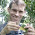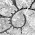## Monday, February 25, 2013

I had a question about the add.random function you posted on your phytools blog a few weeks ago. Occasionally when I add a set of tips, the resulting phylogeny plots so that some branches cross. Anecdotally, it seems to happen only (or at least more often) when the number of added tips > number of ‘real’ tips in the tree (but I haven’t looked at this much). The phylo.object seems to function as I’d expect otherwise so it seems to just be a plotting thing, but I don’t know enough about how plot.phylo reads the phylo object to know for sure.

Indeed this bug is quite easy to replicate:
> set.seed(6)
> tree<-pbtree(n=5)
> layout(c(1,2))

Obviously, there is branch crossing here. I'm going to pass the buck a bit here and mention that although add.random calls the phytools function bind.tip (1,2) internally, bind.tip is little more than a thinly coded wrapper for the 'ape' function bind.tree.

Branch crossing appears to occasionally occur because both plot.phylo (in ape) and plotTree (in phytools) assume a particular ordering for the edges and tips in tree\$edge, which sometimes fails to be satisfied when a lot of tips have been added to the tree. Luckily, this only affects plotting (so far as I know), and none of the other functions of the "phylo" object.

Fortunately, this is also incredibly easy to solve. The following are two different functions that will "untangle" the tree, so to speak:

untangle1<-function(x) reorder(reorder(x,"pruningwise"))
## or

We can try it out on our previous example, from above:
> layout(c(1,2,3))
> plotTree(treeUntangled1)
> plotTree(treeUntangled2)

Since both functions do the job, we can also ask if one is more computationally efficient than the other. Let's do this using a much bigger tree. We'll do that using system.time:
> tree<-pbtree(n=5)
> # this takes a while!
> plotTree(tree,ftype="off",lwd=1)
> # lots of tangles!
> system.time(tree1<-untangle1(tree))
user  system elapsed
0       0       0
> system.time(tree2<-untangle2(tree))
user  system elapsed
0.37    0.00    0.37
> plotTree(tree1,ftype="off",lwd=1)
> # untangled! (tree2 is the same)
> all.equal(tree,tree1)
 TRUE
> all.equal(tree,tree2)
 TRUE

It's a small difference, but the reorder.phylo method is lightning fast! One important note - users should be aware that the tip & node numbers of our tree change when we untangle it.

That's it. I will write a new utility function, untangle, that will do this and also untangle SIMMAP style "phylo" objects. When I get around to that, I'll post it.

1.OK - let me amend my caveat. It seems that for the reorder.phylo method, the node numbers and the order of tip.label is constant; however for the read.tree(write.tree(...)) method, the order and node numbers can change.

2.I can't remember the exact circumstances, but I know I've found instances where reorder.phylo wasn't enough and using the read.tree(write.tree()) trick was necessary.

1.Thanks Dave.

Cool. Yes, I just wrote a utility function that I'll post shortly in which the user can choose either option.

One thing that I discovered, though, is that reorder.phylo(...,"cladewise") does not untangle crossing branches even when reorder(reorder(...,"pruningwise"),"cladewise") does.

More later.

3.More on this here. - Liam

Note: due to the very large amount of spam, all comments are now automatically submitted for moderation.